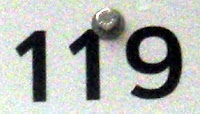## Wednesday, April 28, 2010

### 119

119 = 7 x 17. It is the product of the first two primes ending with 7.

119 = 14 + 15 + 16 + 17 + 18 + 19 + 20.

119 is 1110111 in base 2 (binary) and 77 in base 16.

119 is a number that cannot be written as a sum of three squares.

119 is the smallest three-digit number having two prime digit permutations: 191 and 911.In the United States, the largest amount of money one can have in coins without being able to make change for a dollar is 119 cents. The combination is three quarters, four dimes, and four pennies.